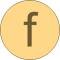# calculator

Interpreter to evaluate simple expressions consisting of +, -, *, /, (), sin(), cos(), tan(), sqrt(), asin(), acos(), atan(), exp(), log(), pi# Information

This information is part of the Modelica Standard Library maintained by the Modelica Association.

#### Syntax

```result = calculator(expression);
```

#### Description

This function demonstrates how a simple expression calculator can be implemented in form of a recursive decent parser using basically the Strings.scanToken(..) and Strings.scanDelimiter(..) function.

The following operations are supported (pi=3.14.. is a predefined constant):

```   +, -
*, /
(expression)
sin(expression)
cos(expression)
tan(expression)
sqrt(expression)
asin(expression)
acos(expression)
atan(expression)
exp(expression)
log(expression)
pi
```

#### Example

```  calculator("2+3*(4-1)");  // returns 11
calculator("sin(pi/6)");  // returns 0.5
```

# Syntax

result = calculator(string)

# Inputs (1)

string Type: String Description: Expression that is evaluated

# Outputs (1)

result Type: Real Description: Value of expression